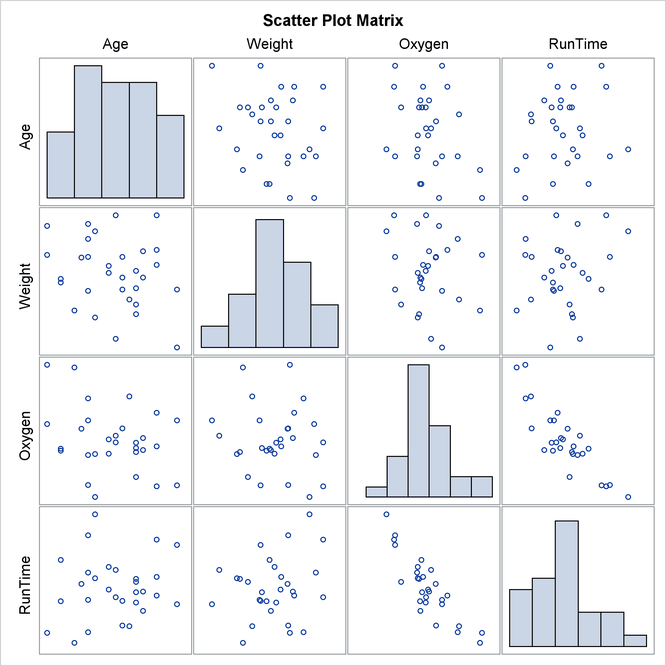# The CORR Procedure

## Getting Started: CORR Procedure

The following statements create the data set `Fitness`, which has been altered to contain some missing values:

```*----------------- Data on Physical Fitness -----------------*
| These measurements were made on men involved in a physical |
| fitness course at N.C. State University.                   |
| The variables are Age (years), Weight (kg),                |
| Runtime (time to run 1.5 miles in minutes), and            |
| Oxygen (oxygen intake, ml per kg body weight per minute)   |
| Certain values were changed to missing for the analysis.   |
*------------------------------------------------------------*;
data Fitness;
input Age Weight Oxygen RunTime @@;
datalines;
44 89.47 44.609 11.37    40 75.07 45.313 10.07
44 85.84 54.297  8.65    42 68.15 59.571  8.17
38 89.02 49.874   .      47 77.45 44.811 11.63
40 75.98 45.681 11.95    43 81.19 49.091 10.85
44 81.42 39.442 13.08    38 81.87 60.055  8.63
44 73.03 50.541 10.13    45 87.66 37.388 14.03
45 66.45 44.754 11.12    47 79.15 47.273 10.60
54 83.12 51.855 10.33    49 81.42 49.156  8.95
51 69.63 40.836 10.95    51 77.91 46.672 10.00
48 91.63 46.774 10.25    49 73.37   .    10.08
57 73.37 39.407 12.63    54 79.38 46.080 11.17
52 76.32 45.441  9.63    50 70.87 54.625  8.92
51 67.25 45.118 11.08    54 91.63 39.203 12.88
51 73.71 45.790 10.47    57 59.08 50.545  9.93
49 76.32   .      .      48 61.24 47.920 11.50
52 82.78 47.467 10.50
;
```

The following statements invoke the CORR procedure and request a correlation analysis:

```ods graphics on;
proc corr data=Fitness plots=matrix(histogram);
run;
```

The "Simple Statistics" table in Figure 2.1 displays univariate statistics for the analysis variables.

Figure 2.1: Univariate Statistics

The CORR Procedure

4 Variables: Age Weight Oxygen RunTime

Simple Statistics
Variable N Mean Std Dev Sum Minimum Maximum
Age 31 47.67742 5.21144 1478 38.00000 57.00000
Weight 31 77.44452 8.32857 2401 59.08000 91.63000
Oxygen 29 47.22721 5.47718 1370 37.38800 60.05500
RunTime 29 10.67414 1.39194 309.55000 8.17000 14.03000

By default, all numeric variables not listed in other statements are used in the analysis. Observations with nonmissing values for each variable are used to derive the univariate statistics for that variable.

The "Pearson Correlation Coefficients" table in Figure 2.2 displays the Pearson correlation, the p-value under the null hypothesis of zero correlation, and the number of nonmissing observations for each pair of variables.

Figure 2.2: Pearson Correlation Coefficients

Pearson Correlation Coefficients
Prob > |r| under H0: Rho=0
Number of Observations
Age Weight Oxygen RunTime
Age
 1.00000 31
 -0.23354 0.2061 31
 -0.31474 0.0963 29
 0.14478 0.4536 29
Weight
 -0.23354 0.2061 31
 1.00000 31
 -0.15358 0.4264 29
 0.20072 0.2965 29
Oxygen
 -0.31474 0.0963 29
 -0.15358 0.4264 29
 1.00000 29
 -0.86843 <.0001 28
RunTime
 0.14478 0.4536 29
 0.20072 0.2965 29
 -0.86843 <.0001 28
 1.00000 29

By default, Pearson correlation statistics are computed from observations with nonmissing values for each pair of analysis variables. Figure 2.2 displays a correlation of –0.86843 between `Runtime` and `Oxygen`, which is significant with a p-value less than 0.0001. That is, there exists an inverse linear relationship between these two variables. As `Runtime` (time to run 1.5 miles in minutes) increases, `Oxygen` (oxygen intake, ml per kg body weight per minute) decreases.

When you use the PLOTS=MATRIX(HISTOGRAM) option, the CORR procedure displays a symmetric matrix plot for the analysis variables in Figure 2.3. The histograms for these analysis variables are also displayed on the diagonal of the matrix plot. This inverse linear relationship between the two variables, `Oxygen` and `Runtime`, is also shown in the plot.

Note that ODS Graphics must be enabled and you must specify the PLOTS= option to produce graphs. For more information about ODS Graphics, see Chapter 21: Statistical Graphics Using ODS in SAS/STAT 14.2 User's Guide.

Figure 2.3: Symmetric Matrix Plot